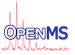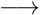OpenMS  2.7.0
MapRTTransformer

Applies retention time transformations to maps.

 potential predecessor toolsMapRTTransformerpotential successor tools MapAlignerIdentification (or another alignment algorithm) FeatureLinkerUnlabeled or FeatureLinkerUnlabeledQT

This tool can apply retention time transformations to different types of data (mzML, featureXML, consensusXML, and idXML files). The transformations might have been generated by a previous invocation of one of the MapAligner tools (linked below). However, the trafoXML file format is not very complicated, so it is relatively easy to write (or generate) your own files. Each input file will give rise to one output file.

MapAlignerIdentification MapAlignerPoseClustering MapAlignerSpectrum

With this tool it is also possible to invert transformations, or to fit a different model than originally specified to the retention time data in the transformation files. To fit a new model, choose a value other than "none" for the model type (see below).

Original retention time values can be kept as meta data. With the option `store_original_rt`, meta values with the name "original_RT" and the original retention time will be created for every major data element (spectrum, chromatogram, feature, consensus feature, peptide identification), unless they already exist - "original_RT" values from a previous invocation will not be overwritten.

Since OpenMS 1.8, the extraction of data for the alignment has been separate from the modeling of RT transformations based on that data. It is now possible to use different models independently of the chosen algorithm. The different available models are:

The following parameters control the modeling of RT transformations (they can be set in the "model" section of the INI file):

NameTypeDefaultRestrictionsDescription
type stringnone none, linear, b_spline, lowess, interpolatedType of model
linear:symmetric_regression stringfalse true, falsePerform linear regression on 'y - x' vs. 'y + x', instead of on 'y' vs. 'x'.
linear:x_weight string 1/x, 1/x2, ln(x), Weight x values
linear:y_weight string 1/y, 1/y2, ln(y), Weight y values
linear:x_datum_min float1.0e-15  Minimum x value
linear:x_datum_max float1.0e15  Maximum x value
linear:y_datum_min float1.0e-15  Minimum y value
linear:y_datum_max float1.0e15  Maximum y value
b_spline:wavelength float0.0 min: 0.0Determines the amount of smoothing by setting the number of nodes for the B-spline. The number is chosen so that the spline approximates a low-pass filter with this cutoff wavelength. The wavelength is given in the same units as the data; a higher value means more smoothing. '0' sets the number of nodes to twice the number of input points.
b_spline:num_nodes int5 min: 0Number of nodes for B-spline fitting. Overrides 'wavelength' if set (to two or greater). A lower value means more smoothing.
b_spline:extrapolate stringlinear linear, b_spline, constant, global_linearMethod to use for extrapolation beyond the original data range. 'linear': Linear extrapolation using the slope of the B-spline at the corresponding endpoint. 'b_spline': Use the B-spline (as for interpolation). 'constant': Use the constant value of the B-spline at the corresponding endpoint. 'global_linear': Use a linear fit through the data (which will most probably introduce discontinuities at the ends of the data range).
b_spline:boundary_condition int2 min: 0 max: 2Boundary condition at B-spline endpoints: 0 (value zero), 1 (first derivative zero) or 2 (second derivative zero)
lowess:span float0.666666666666667 min: 0.0 max: 1.0Fraction of datapoints (f) to use for each local regression (determines the amount of smoothing). Choosing this parameter in the range .2 to .8 usually results in a good fit.
lowess:num_iterations int3 min: 0Number of robustifying iterations for lowess fitting.
lowess:delta float-1.0  Nonnegative parameter which may be used to save computations (recommended value is 0.01 of the range of the input, e.g. for data ranging from 1000 seconds to 2000 seconds, it could be set to 10). Setting a negative value will automatically do this.
lowess:interpolation_type stringcspline linear, cspline, akimaMethod to use for interpolation between datapoints computed by lowess. 'linear': Linear interpolation. 'cspline': Use the cubic spline for interpolation. 'akima': Use an akima spline for interpolation
lowess:extrapolation_type stringfour-point-linear two-point-linear, four-point-linear, global-linearMethod to use for extrapolation outside the data range. 'two-point-linear': Uses a line through the first and last point to extrapolate. 'four-point-linear': Uses a line through the first and second point to extrapolate in front and and a line through the last and second-to-last point in the end. 'global-linear': Uses a linear regression to fit a line through all data points and use it for interpolation.
interpolated:interpolation_type stringcspline linear, cspline, akimaType of interpolation to apply.
interpolated:extrapolation_type stringtwo-point-linear two-point-linear, four-point-linear, global-linearType of extrapolation to apply: two-point-linear: use the first and last data point to build a single linear model, four-point-linear: build two linear models on both ends using the first two / last two points, global-linear: use all points to build a single linear model. Note that global-linear may not be continuous at the border.

Note
As output options, either `out` or `trafo_out` has to be provided. They can be used together.
Currently mzIdentML (mzid) is not directly supported as an input/output format of this tool. Convert mzid files to/from idXML using IDFileConverter if necessary.

The command line parameters of this tool are:

```MapRTTransformer -- Applies retention time transformations to maps.
Full documentation: http://www.openms.de/doxygen/release/2.7.0/html/TOPP_MapRTTransformer.html
Version: 2.7.0 Sep 13 2021, 20:58:47, Revision: 9110e58
To cite OpenMS:
Rost HL, Sachsenberg T, Aiche S, Bielow C et al.. OpenMS: a flexible open-source software platform for mass spectrometry data analysis. Nat Meth. 2016; 13, 9: 741-748. doi:10.1038/nmeth.3959.

Usage:
MapRTTransformer <options>

This tool has algorithm parameters that are not shown here! Please check the ini file for a detailed descript
ion or use the --helphelp option.

Options (mandatory options marked with '*'):
-in <file>           Input file to transform (separated by blanks) (valid formats: 'mzML', 'featureXML',
'consensusXML', 'idXML')
-out <file>          Output file (same file type as 'in'). This option or 'trafo_out' has to be provided;
they can be used together. (valid formats: 'mzML', 'featureXML', 'consensusXML', 'idXM
L')
-trafo_in <file>*    Transformation to apply (valid formats: 'trafoXML')
-trafo_out <file>    Transformation output file. This option or 'out' has to be provided; they can be used
together. (valid formats: 'trafoXML')
-invert              Invert transformation (approximatively) before applying it
-store_original_rt   Store the original retention times (before transformation) as meta data in the output
file

Common TOPP options:
-ini <file>          Use the given TOPP INI file
-threads <n>         Sets the number of threads allowed to be used by the TOPP tool (default: '1')
-write_ini <file>    Writes the default configuration file
--help               Shows options
--helphelp           Shows all options (including advanced)

The following configuration subsections are valid:
- model   Options to control the modeling of retention time transformations from data

You can write an example INI file using the '-write_ini' option.
Documentation of subsection parameters can be found in the doxygen documentation or the INIFileEditor.
- http://www.openms.de/doxygen/release/2.7.0/html/TOPP_MapRTTransformer.html

```

INI file documentation of this tool:

Legend:
required parameter
+MapRTTransformerApplies retention time transformations to maps.
version2.7.0 Version of the tool that generated this parameters file.
++1Instance '1' section for 'MapRTTransformer'
in Input file to transform (separated by blanks)input file*.mzML,*.featureXML,*.consensusXML,*.idXML
out Output file (same file type as 'in'). This option or 'trafo_out' has to be provided; they can be used together.output file*.mzML,*.featureXML,*.consensusXML,*.idXML
trafo_in Transformation to applyinput file*.trafoXML
trafo_out Transformation output file. This option or 'out' has to be provided; they can be used together.output file*.trafoXML
invertfalse Invert transformation (approximatively) before applying ittrue,false
store_original_rtfalse Store the original retention times (before transformation) as meta data in the output filetrue,false
log Name of log file (created only when specified)
debug0 Sets the debug level
threads1 Sets the number of threads allowed to be used by the TOPP tool
no_progressfalse Disables progress logging to command linetrue,false
forcefalse Overrides tool-specific checkstrue,false
testfalse Enables the test mode (needed for internal use only)true,false
+++modelOptions to control the modeling of retention time transformations from data
typenone Type of modelnone,linear,b_spline,lowess,interpolated
++++linearParameters for 'linear' model
symmetric_regressionfalse Perform linear regression on 'y - x' vs. 'y + x', instead of on 'y' vs. 'x'.true,false
x_weight Weight x values1/x,1/x2,ln(x),
y_weight Weight y values1/y,1/y2,ln(y),
x_datum_min1.0e-15 Minimum x value
x_datum_max1.0e15 Maximum x value
y_datum_min1.0e-15 Minimum y value
y_datum_max1.0e15 Maximum y value
++++b_splineParameters for 'b_spline' model
wavelength0.0 Determines the amount of smoothing by setting the number of nodes for the B-spline. The number is chosen so that the spline approximates a low-pass filter with this cutoff wavelength. The wavelength is given in the same units as the data; a higher value means more smoothing. '0' sets the number of nodes to twice the number of input points.0.0:∞
num_nodes5 Number of nodes for B-spline fitting. Overrides 'wavelength' if set (to two or greater). A lower value means more smoothing.0:∞
extrapolatelinear Method to use for extrapolation beyond the original data range. 'linear': Linear extrapolation using the slope of the B-spline at the corresponding endpoint. 'b_spline': Use the B-spline (as for interpolation). 'constant': Use the constant value of the B-spline at the corresponding endpoint. 'global_linear': Use a linear fit through the data (which will most probably introduce discontinuities at the ends of the data range).linear,b_spline,constant,global_linear
boundary_condition2 Boundary condition at B-spline endpoints: 0 (value zero), 1 (first derivative zero) or 2 (second derivative zero)0:2
++++lowessParameters for 'lowess' model
span0.666666666666667 Fraction of datapoints (f) to use for each local regression (determines the amount of smoothing). Choosing this parameter in the range .2 to .8 usually results in a good fit.0.0:1.0
num_iterations3 Number of robustifying iterations for lowess fitting.0:∞
delta-1.0 Nonnegative parameter which may be used to save computations (recommended value is 0.01 of the range of the input, e.g. for data ranging from 1000 seconds to 2000 seconds, it could be set to 10). Setting a negative value will automatically do this.
interpolation_typecspline Method to use for interpolation between datapoints computed by lowess. 'linear': Linear interpolation. 'cspline': Use the cubic spline for interpolation. 'akima': Use an akima spline for interpolationlinear,cspline,akima
extrapolation_typefour-point-linear Method to use for extrapolation outside the data range. 'two-point-linear': Uses a line through the first and last point to extrapolate. 'four-point-linear': Uses a line through the first and second point to extrapolate in front and and a line through the last and second-to-last point in the end. 'global-linear': Uses a linear regression to fit a line through all data points and use it for interpolation.two-point-linear,four-point-linear,global-linear
++++interpolatedParameters for 'interpolated' model
interpolation_typecspline Type of interpolation to apply.linear,cspline,akima
extrapolation_typetwo-point-linear Type of extrapolation to apply: two-point-linear: use the first and last data point to build a single linear model, four-point-linear: build two linear models on both ends using the first two / last two points, global-linear: use all points to build a single linear model. Note that global-linear may not be continuous at the border.two-point-linear,four-point-linear,global-linear